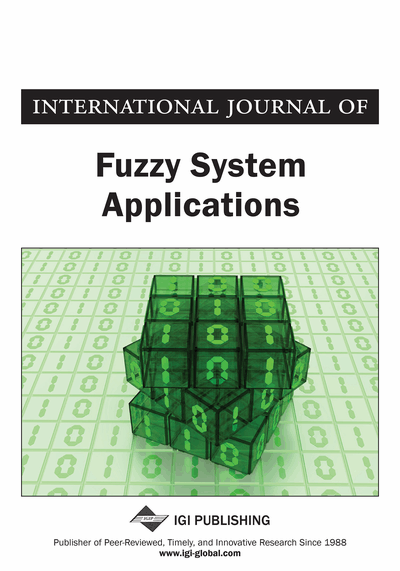# Rough Fuzzy Automata and Rough Fuzzy Grammar

Kanchan Tyagi, Alka Tripathi
DOI: 10.4018/IJFSA.2017010103
OnDemand:
(Individual Articles)
Available
\$37.50
No Current Special Offers

## Abstract

Automata theory plays a key role in computational theory as many computational problems can be solved with its help. Formal grammar is a special type of automata designed for linguistic purposes. Formal grammar generates formal languages. Rough grammar and rough languages were introduced to incorporate the imprecision of real languages in formal languages. These languages have limitations on uncertainty. The authors have considered both uncertainty and approximations to define rough fuzzy grammar and rough fuzzy languages. Under certain restrictions, their grammar reduces to formal grammar. Furthermore, the authors have proposed definition of rough fuzzy automata that accepts rough fuzzy regular language.
Article Preview
Top

## Introduction

Fuzzy set theory and rough set theory are model of uncertainty and approximations. The notion of fuzzy set theory and rough set theory were introduced by Zadeh (1965) and Pawlak (1982). The Fuzzy set theory addresses the definition of vagueness boundary of a class that is a generalization of set characteristics functions and the rough set theory acts as indiscernibility between objects, which is distinctively characterized by equivalence relation. The idea of rough set is based on approximations of sets and these approximations are defined using the equivalence classes. Dubois et al. (1990) gave idea of generalized version of rough set which is called as rough fuzzy set. Recent extensions of rough set theory have developed a new method for decomposition of large data sets. The rough set methodologies have found many applications.

Two basic fuzzy automata models, Mealy and Moore types for lexical analysis were introduced by Salomaa et al. (1995). Finite state automata are significantly used in many areas. Malik & Modersan (2002) proposed fuzzy language, fuzzy grammar and their applications. Basu (2005) introduced rough finite state automata. The difference between rough automata and other automata is of their transition map. Tiwari et al. (2014) introduced the rough finite state machine with output. Tripathi et al. (2014) proposed approximate equalities of rough set using interior and closure (rough equality, rough equivalence, approximate rough equality and approximate rough equivalence). We found that rough equivalence is better in real life situation than others.

Noam Chomsky gave a mathematical model of a grammar. W. G Wee introduced the concept of fuzzy grammar and fuzzy language. Grammars generate the languages. If the grammar is regular, then we can define rough fuzzy regular grammar. Concept of fuzzy language was introduced by (Lee, 1969). Fuzzy Language is defined with fuzzy subsets of strings and these strings are made from finite alphabets. In fuzzy regular language, strings are finite and regular with membership values. The concept of rough language was introduced by G. Paun et al. (1998). Rough grammar and rough languages were introduced by Basu (2003). Rough fuzzy regular grammar defined as ordered pairs of lower and upper approximations with membership values.

Intuitionistic fuzzy sets are based on membership and nonmember ship values. Nan et al. (2014) extended a method for matrix solving games with payoffs of trapezoidal intuitionistic fuzzy numbers. The validity of proposed method is illustrated with numerical example. Interval-valued intuitionistic fuzzy (IVIF) sets are a valuable device to deal with uncertainty inherent in decision data and decision making process. Wang et al. (2013) extended a methodology for solving multiattribute decision making (MADM) with both ratings of alternatives on attributes and weights being expressed with IVIF sets. Li et al. (2013) extended new fuzzy linear programming method for solving such MADM problems. The book by Li et al. (2014) has practical applications in decision science, game theory, economics etc.

## Complete Article List

Search this Journal:
Reset
Volume 13: 1 Issue (2024)
Volume 12: 1 Issue (2023)
Volume 11: 4 Issues (2022)
Volume 10: 4 Issues (2021)
Volume 9: 4 Issues (2020)
Volume 8: 4 Issues (2019)
Volume 7: 4 Issues (2018)
Volume 6: 4 Issues (2017)
Volume 5: 4 Issues (2016)
Volume 4: 4 Issues (2015)
Volume 3: 4 Issues (2013)
Volume 2: 4 Issues (2012)
Volume 1: 4 Issues (2011)
View Complete Journal Contents Listing